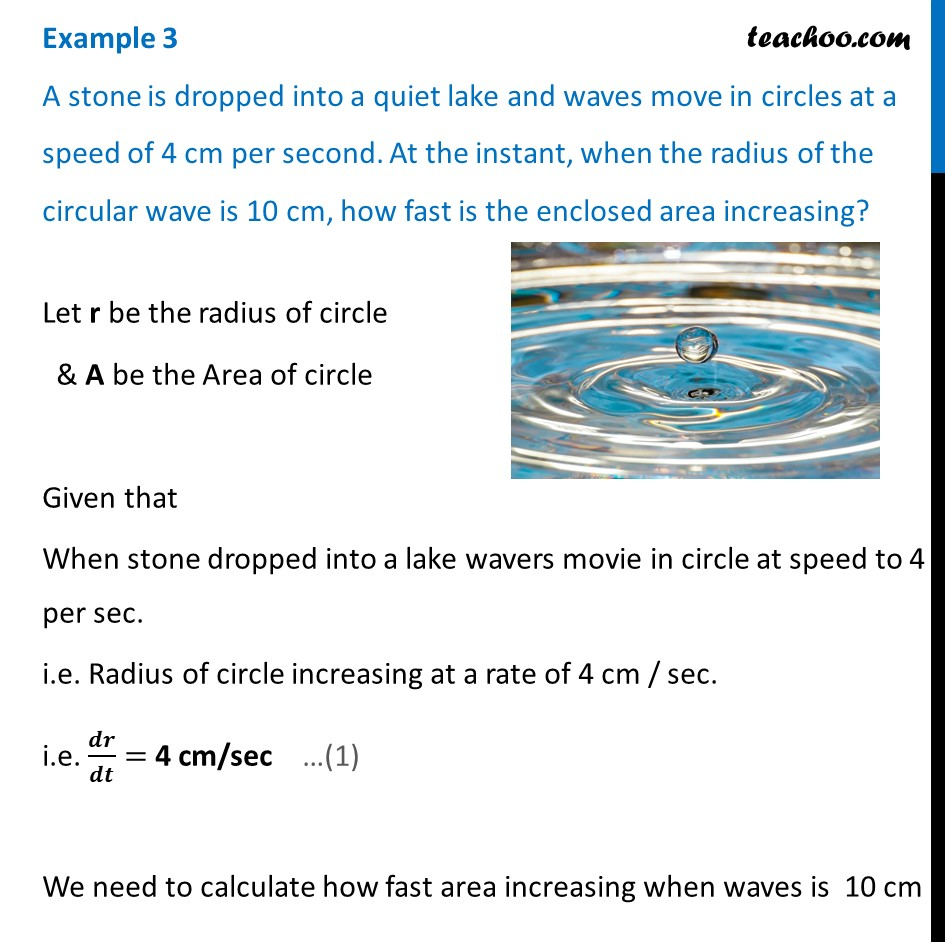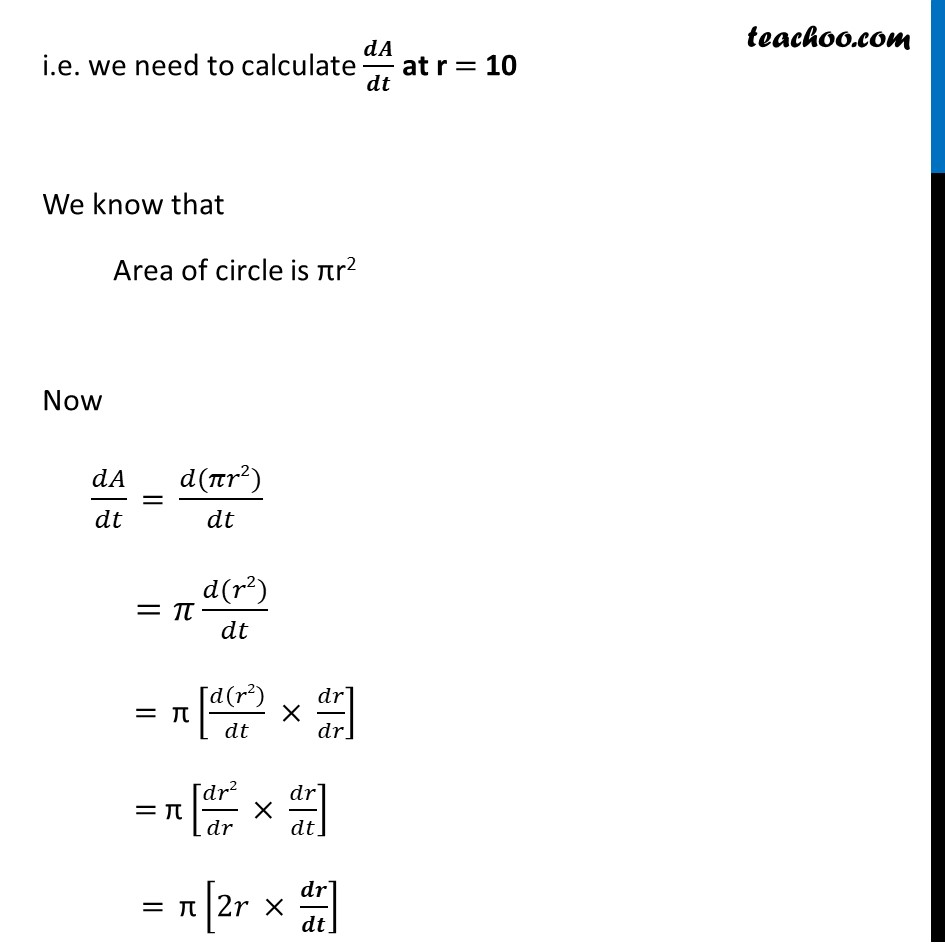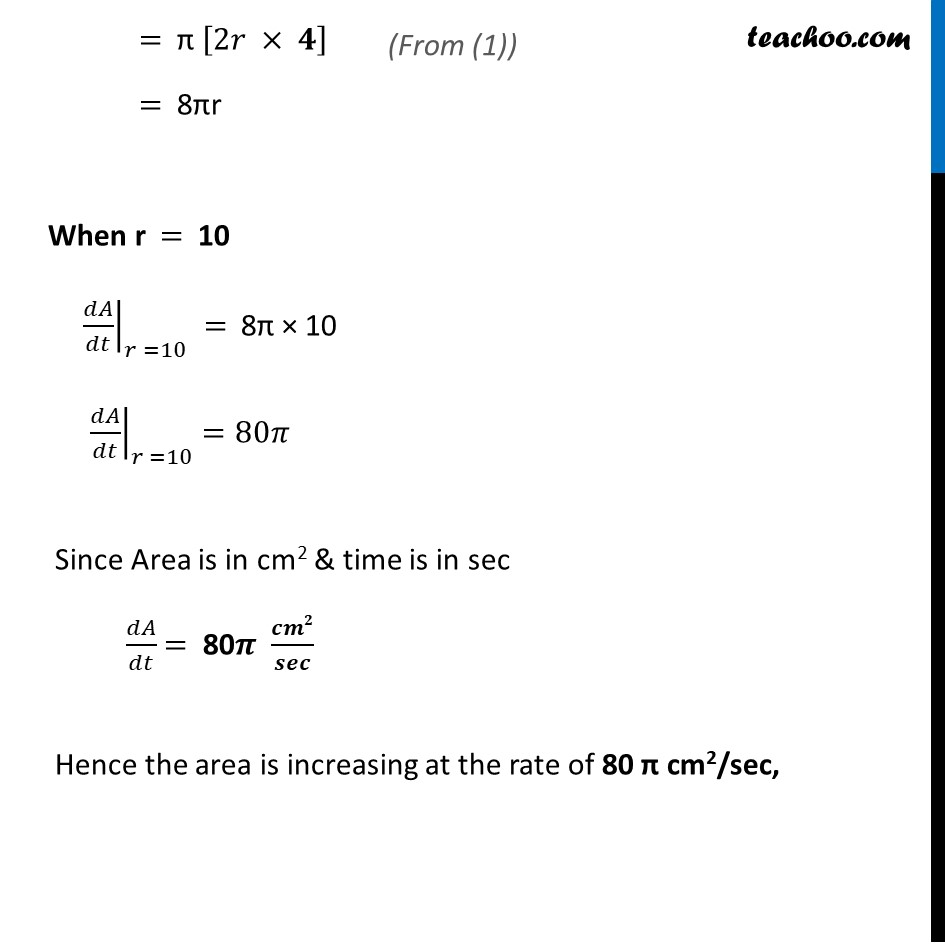Examples

Chapter 6 Class 12 Application of Derivatives
Serial order wiseLearn in your speed, with individual attention - Teachoo Maths 1-on-1 Class

### Transcript

Example 3 A stone is dropped into a quiet lake and waves move in circles at a speed of 4 cm per second. At the instant, when the radius of the circular wave is 10 cm, how fast is the enclosed area increasing?Let r be the radius of circle & A be the Area of circle Given that When stone dropped into a lake wavers movie in circle at speed to 4 cm per sec. i.e. Radius of circle increasing at a rate of 4 cm / sec. i.e. 𝒅𝒓/𝒅𝒕 = 4 cm/sec We need to calculate how fast area increasing when waves is 10 cm i.e. we need to calculate 𝒅𝑨/𝒅𝒕 at r = 10 We know that Area of circle is πr2 Now 𝑑𝐴/𝑑𝑡 = (𝑑(𝜋𝑟2))/𝑑𝑡 =𝜋 (𝑑(𝑟2))/𝑑𝑡 = π [𝑑(𝑟2)/𝑑𝑡 × 𝑑𝑟/𝑑𝑟] = π [𝑑𝑟2/𝑑𝑟 × 𝑑𝑟/𝑑𝑡] = π [2𝑟 × 𝒅𝒓/𝒅𝒕] = π [2𝑟 × 𝟒] = 8πr When r = 10 ├ 𝑑𝐴/𝑑𝑡┤|_(𝑟 =10) = 8π × 10 ├ 𝑑𝐴/𝑑𝑡┤|_(𝑟 =10) =80𝜋 Since Area is in cm2 & time is in sec 𝑑𝐴/𝑑𝑡 = 80𝝅 𝒄𝒎𝟐/𝒔𝒆𝒄 Hence the area is increasing at the rate of 80 π cm2/sec,